0
163

# Verbal Reasoning Questions for MAH-CET

Question 1: ‘Music’ is related to ‘Notes’ in the same way as ‘Language’ is related to………………

a) Sentences

b) Combination

c) Grammar

d) Alphabets

e) Syntax

Solution:

Music is composed from notes, similarly a language is made from sentences.

=> Ans – (A)

Question 2: if’ AxB’ means B is the father of A and ‘A+B’ means A is the wife of B and A /B means A is the brother of B , then what is the relation of J with L in the ‘ J + H / R x L ‘.

a) Daughter

b) Daughter in law

c) Sister in law

d) Cannot determined

e) None of these

Solution:

Expression : J + H / R x L

J is the wife of H, and H is the brother of R.

Also, L is the father of R, => H is the son of L.

Thus, J is the daughter-in-law of L.

=> Ans – (B)

Instructions

Study the following information carefully and answer the following the questions

Eight friends J, K, L, M, N, O, P and Q are sitting around a circle table facing the center. J is not the neighbour of N. L is the third to right of K. Q is second to the left of N, who is the next to the right of L. O is not the neighbour of N or K and is to the immediate left of the P.

Question 3: Who is to immediate left of Q?

a) P

b) L

c) Q

d) J

e) None of these

Solution:

L is the third to right of K and N is the next to the right of L

=> N is sitting opposite to K.

Also, Q is second to the left of N, => Q is sitting second to the right of K and to the immediate left of L.

O is not the neighbour of N or K, => O is sitting opposite Q and second to the left of K.

Since, J is not the neighbor of N, => J is sitting between Q and K.

The only empty position is taken by M. The arrangement is :

Clearly, J is to the immediate left of Q.

=> Ans – (D)

Question 4: Who is to the immediate right of K

a) J

b) P

c) Q

d) Cannot determined

e) None of these

Solution:

L is the third to right of K and N is the next to the right of L

=> N is sitting opposite to K.

Also, Q is second to the left of N, => Q is sitting second to the right of K and to the immediate left of L.

O is not the neighbour of N or K, => O is sitting opposite Q and second to the left of K.

Since, J is not the neighbor of N, => J is sitting between Q and K.

The only empty position is taken by M. The arrangement is :

J is to the immediate right of K.

=> Ans – (A)

Question 5: Which of the following groups of has the first person sitting between the other two persons

a) PKJ

b) LQJ

c) QNL

d) LMN

e) None of these

Solution:

L is the third to right of K and N is the next to the right of L

=> N is sitting opposite to K.

Also, Q is second to the left of N, => Q is sitting second to the right of K and to the immediate left of L.

O is not the neighbour of N or K, => O is sitting opposite Q and second to the left of K.

Since, J is not the neighbor of N, => J is sitting between Q and K.

The only empty position is taken by M. The arrangement is :

(A) : P does not sit between K & J.

(B) : L does not sit between Q & J.

(C) : Q does not sit between N & L.

(D) : L does not sit between M & N.

=> Ans – (E)

Take Free MAH-CET mock tests here

Question 6: Which of the following pair of persons represents O ‘s neighbours?

a) L&N;

b) P&K;

c) M&P;

d) N&P;

e) None of these

Solution:

L is the third to right of K and N is the next to the right of L

=> N is sitting opposite to K.

Also, Q is second to the left of N, => Q is sitting second to the right of K and to the immediate left of L.

O is not the neighbour of N or K, => O is sitting opposite Q and second to the left of K.

Since, J is not the neighbor of N, => J is sitting between Q and K.

The only empty position is taken by M. The arrangement is :

M & P are O’s neighbours.

=> Ans – (C)

Question 7: Which of the following is the correct position of L?

a) To the immediate right of the N

b) To the immediate right of Q

c) To the immediate LEFT of the J

d) To the immediate LEFT of the Q

e) None of these

Solution:

L is the third to right of K and N is the next to the right of L

=> N is sitting opposite to K.

Also, Q is second to the left of N, => Q is sitting second to the right of K and to the immediate left of L.

O is not the neighbour of N or K, => O is sitting opposite Q and second to the left of K.

Since, J is not the neighbor of N, => J is sitting between Q and K.

The only empty position is taken by M. The arrangement is :

Clearly, L is to the immediate right of Q.

=> Ans – (B)

Question 8: which of the following values satisfy the inequality ?
P (P-3) < 4P- 12 ?

a) p>4 or p<3

b) 4<=P<7

c) P>3,P<=5

d) 3

e) P=4,P=-3

Solution:

Expression : $P(P-3)<4P-12$

=> $P^2-3P-4P+12<0$

=> $P^2-7P+12<0$

=> $(P-3)(P-4)<0$

=> $P<3$ and $P>4$

=> Ans – (A)

Question 9: If $means ‘plus (+), # means ‘minus (-), @ means ‘multiplied (x) and * means ‘divided ($\div$), then what is the value of ’16$ 4 @ 5 # 72 * 8’ ?

a) 29

b) 27

c) 25

d) 36

e) None of these

Solution:

Expression : ’16 $4 @ 5 # 72 * 8’ ?$\equiv16+4\times5-72\div8$=$16+20-\frac{72}{8}$=$36-9=27$=> Ans – (B) Question 10: How many meaningful english words can be formed from the letters ‘’ACER ‘’ using each letter once a) None b) One c) Two d) Three e) More than three 10) Answer (D) Solution: We can form 3 words from the given word “ACER” i.e CARE, RACE, ACRE. Hence, option D is the correct answer. Question 11: How many such pairs of letters are there in the word SCHEDULE (in both forward and backward directions),each of which has as many letters between them in the word as in the English alphabetical series? a) None b) One c) Two d) Three e) More than three 11) Answer (D) Solution: Word – SCHEDULE There are 3 pairs of letters which have as many letters between them in the word as in the English alphabetical series. = (C,E) , (E,D) , (H,L) => Ans – (D) Question 12: If ‘sky’ means ‘star’; ‘star’ means ‘cloud’; ‘cloud’ means ‘earth’. ‘earth’ means ‘tree’ and ‘tree’ means ‘book’, then where do the birds fly? a) sky b) star c) cloud d) Data inadequate e) None of these 12) Answer (B) Solution: Birds fly in the sky, but it is given that ‘sky’ means ‘star’ Thus, birds fly in star. => Ans – (B) Instructions In each question below is given a statement followed by two assumptions numbered I and II. An assumption is something supposed or taken for granted. You have to consider the statement and the following assumptions is implicit in the statement. Question 13: Statement: The state government has issued a notification to the effect that all the coaching classes must register themselves immediately giving details of fees, infrastructural facilities and bio-data of teachers. Assumptions: I. All those coaching classes which do not have requisite infrastructure and teachers may close down II. All the coaching classes may hereafter charge uniform fees for all the students a) Give answer A if only assumption I is implicit b) Give answer B if only assumption II is implicit c) Give answer C if either assumption I or II is implicit d) Give answer D if neither assumption I nor II is implicit e) Give answer E if both assumptions I nor II is implicit 13) Answer (A) Solution: Statement II is not an implicit assumption. It is not necessary that after this notice the coaching will charge uniformly. Fees is dependent on many factors such as material provided, infrastructure quality, teacher quality, number of dedicated hours etc. It is not necessary that all these factors are same due to the notice. Statement I is an implicit assumption. If government issues this notice, it may be the case that coaching which have poor teachers and infrastructure may close down. Thus, A is the correct answer. Instructions In making decision about important questions, it is desirable to be able to distinguish between ‘strong’ arguments and ‘weak’ arguments so far as they relate to the question. Strong arguments are those which are are of major importance and directly related to the question. Weak arguments are those which are minor importance and also be directly related to the question of may be related to a trivial aspect of the question. After each question two Argument I and II are given. You have to decide which argument is strong and which argument is weak. Question 14: Should India make an all out effort to secure permanent membership of UN Security Council at any cost ? Arguments: I. Yes, this is the only way to establish its supremacy in the region. II. No, there are many other urgent issues which need government’s immediate attention which will make life of citizens comfortable. a) Give answer A if only argument I is strong b) Give answer B if only argument II is strong c) Give answer C if either argument I or II is strong d) Give answer D if neither argument I nor II is strong e) Give answer E if both argument I and II are strong 14) Answer (D) Solution: Neither I nor II are important aspect or directly related to the question. The information mentioned in II is not directly related to the argument. So, it is not strong. Similarly, I is also a insignificant aspect of the question. So, it can also not be stated as strong. So, D is the correct answer. Question 15: Should there be only five holidays in a calendar year in all the states of India ? Arguments: I. Yes, we can not afford to lose so many working days as at present. II. Yes, the USA has few holidays in a calendar year. a) Give answer A if only argument I is strong b) Give answer B if only argument II is strong c) Give answer C if either argument I or II is strong d) Give answer D if neither argument I nor II is strong e) Give answer E if both argument I and II are strong 15) Answer (D) Solution: Argument 1 says that we can not afford to lose so many working days as at present. But this is not backed by any reasoning. Thus it is incorrect. Argument 2 says that the USA has few holidays in a calendar year but it does not mention the number of holidays at all. Thus it is also incorrect. Hence Option D. Instructions Each of the questions below consists of a question and two statements numbered I and II given below it. You have to decide whether the data provided in the statements are sufficient to answer the question. Read both the statements and answer the below questions a: if the data in Statement I alone are sufficient to answer the question, while the data in Statement II alone are not sufficient to answer the question. b: if the data in Statement II alone are sufficient to answer the question, while the data in Statement I alone are not sufficient to answer the question. c: if the data either in Statement I alone or in Statement II alone are sufficient to answer the question. d: if the data even in both Statements I and II together are not sufficient to answer the question. e: if the data in both Statements I and II together are necessary to answer the question. Question 16: How is A related to F?I. A is mother of B. D is brother of B. R is father of D. R has one son and one daughter. T is father of R. T is married to F. II. F is married to T. T has only two children R and C. R is married to A. A has two children. C is aunt of B and D. a) if the data in Statement I alone are sufficient to answer the question, while the data in Statement II alone are not sufficient to answer the question. b) if the data in Statement II alone are sufficient to answer the question, while the data in Statement I alone are not sufficient to answer the question. c) if the data either in Statement I alone or in Statement II alone are sufficient to answer the question. d) if the data even in both Statements I and II together are not sufficient to answer the question. e) if the data in both Statements I and II together are necessary to answer the question. 16) Answer (A) Solution: I : A is mother of B. D is brother of B. R is father of D. R has one son and one daughter. => R is husband of A, and B is sister of D. T is father of R. T is married to F. => F is mother of R. Thus, A is daughter-in-law of F, hence I alone is sufficient. II : F is married to T. T has only two children R and C. R is married to A. A has two children. C is aunt of B and D. Thus, A can be either son-in-law or daughter-in-law of F, hence II alone is sufficient.$\therefore$Statement I alone is sufficient. => Ans – (A) Question 17: What is the code for ‘reason’ in a certain code language? I. In that code language ‘little reason to believe’ is coded as ‘& 4$ 2’ and ‘reason is never little’ is coded as ‘3 & 8 2’.
II. In that code language ‘little to reason now’ is coded as ‘& 2 % 4’ and ‘believe now is problem’ is coded as ‘% 8 $5’ a) if the data in Statement I alone are sufficient to answer the question, while the data in Statement II alone are not sufficient to answer the question. b) if the data in Statement II alone are sufficient to answer the question, while the data in Statement I alone are not sufficient to answer the question. c) if the data either in Statement I alone or in Statement II alone are sufficient to answer the question. d) if the data even in both Statements I and II together are not sufficient to answer the question. e) if the data in both Statements I and II together are necessary to answer the question. 17) Answer (D) Solution: I : In that code language ‘little reason to believe’ is coded as ‘& 4$ 2’ and ‘reason is never little’ is coded as ‘3 & 8 2’.

The common words in both inferences are ‘little‘ and ‘reason‘ coded as = ‘&’ or ‘2’

Thus, we cannot find the code for reason, hence I alone is insufficient.

II : In that code language ‘little to reason now’ is coded as ‘& 2 % 4’ and ‘believe now is problem’ is coded as ‘% 8 $5 The only common word is ‘now‘ coded as = ‘%’ Thus, we cannot find the code for reason, hence II alone is insufficient. Similarly, by combining both statements, we still cannot find the code for ‘reason’.$\therefore$Both statements even together are not sufficient. => Ans – (D) Instructions In each question given below a statement is followed by three Courses of action numbered I, II and III. A course of action is a step or administrative decision to be taken for improvement follow up or further action in regard to the problem, policy etc. on the basis of the information given in the statement. You have to assume every thing in the statement to be true, then decide which of the three given/ suggested Courses of action logically follows for pursuing and decide the answer. Question 18: Statement : The chairman of the car company announced in the meeting that all trials of its first product the new car model ‘M’ are over and company plans to launch its car in the market after six months. Courses of action : (I) The network of dealers is to be finalised and all legal, financial and other matters in this connection will have to be finalised shortly. (II) The company will have to make plan for product other than car. (III) Material, managerial and other resources will have to be in fine tune to maintain production schedule a) I and III only b) Only I c) All of three d) Only II e) None of these 18) Answer (A) Solution: Once the trails are over, the best availability of material managerial and other resources is necessary to maintain production schedule. Hence III follows. As mentioned in the statement, ‘model M is its first product’, so it is necessary to finalise the network of dealers and all matters regarding the sale of the product. Hence I follows. II has no connection with the statement. Thus, only I and III follow. => Ans – (A) Question 19: In a row of children facing NORTH Ritesh is twelfth from the left end .Sudhir who is twenty second from right end is fourth to the right of Ritesh . Total how many children are there in the row? a) 35 b) 36 c) 37 d) 34 e) None of these 19) Answer (C) Solution: Ritesh is 12th from the left end. Sudhir is fourth to the right of Ritesh. Hence, Sudhir is 16th from the left end. And also Sudhir is 22nd from the right end. There are 11 people to the left of Ritesh, 21 people to the right of Sudhir and 3 people in between Ritesh and Sudhir. The total number of children’s in the row is: 11 + 3 + 21 + 2(including both Ritesh and Sudhir) = 37. Hence, option C is correct. Question 20: Which of the following figures represents the relation between ‘Sparrows’, ‘Birds’ and ‘Crows’ ? a)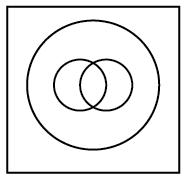b)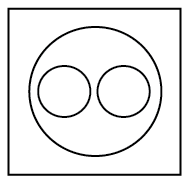c)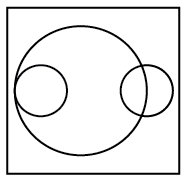d)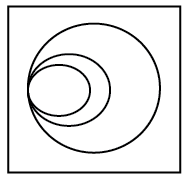e)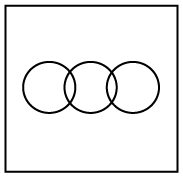20) Answer (B) Solution: Sparrows and crows are entirely different. But, both are birds. => Ans – (B) Question 21: Which of the following symbols should be placed in blank spaces respectively (in the same order from left to right) in order to complete the given expression in such a manner that both ‘W > R as well as ‘E ≥ S’ definitely hold true? W_E_A_R_ S a) >, =, ≥, ≥ b) >, ≥, =, > c) ≤, ≥, =, ≥ d) ≤, =, >, ≥ e) ≥, <, ≤, = 21) Answer (A) Solution: Given :$W>R$and$E \geq S$Now, for above statements to be true, the expression :$W>E=A\geq R\geq S$=> Ans – (A) Question 22: Four of the following five are like in a certain code ,which one does not belongs to that group? a) ROSE b) JASMINE c) HIBISCUS d) MARIGOLD e) LOTUS 22) Answer (E) Solution: Here, all except Lotus are flowers which grow on land while lotus is a water flower. => Ans – (E) Question 23: Study the following information carefully and answer the question which follows : Farmers should use hydroponics for farming. Hydroponics is growing plants in liquid nutrient solution. Since it does not require soil, farmers can save a large amount of time by avoiding tilling and de-weeding of their fields. Which of the following statements does not weaken the given statement? a) Initial set up cost for hydroponics is very high as the necessary equipment and inputs are three times more expensive than that used in traditional methods. b) Disease causing micro-organisms that are water-based can enter and subsist much more easily in hydroponics based environment as compared to traditional farming. c) Rather than using only hydroponics or only traditional farming, most of the farmers use both simultaneously. d) Without soil to serve as a buffer, if the hydroponics system fails, plant death occurs very rapidly leading to loss of 70-85% crop. e) The production using hydroponics is very limited and is more efficient for house gardens rather than large scale farming. 23) Answer (C) Solution: The given statement supports the use of hydroponics among farmers. The first option definitely weakens the statement as it mentions the high cost of hydroponics. Similarly, the second option also states the disease caused by the water used in hydroponics, it also weakens the given statement. The fourth option mentions about the loss of crop due to this method and the final option talks about its limitations, thus both weakening the statement. But the third option gives a choice for the farmers to use hydroponics and thus does not weaken the given statement. => Ans – (C) Question 24: If it is possible to form a number with the first, the fourth and the seventh digits of the number 4671358, which is the perfect square of a two-digit odd number, which of the following will be the digit in the tenth place of that two digit odd number ? If no such number can be formed, give ‘O’ as the answer and if more than one such number can be made, give ‘X’ as the answer. a) 2 b) 9 c) 3 d) O e) X 24) Answer (A) Solution: Number – 4671358 1st, 4th and 7th digits = 4, 1, 8 Now, if a number should be a perfect square of an odd number, then the number should also be odd, i.e. end with ‘1’ in this case. Numbers ending with 1 = 481, 841 Now,$841=(29)^2$and 481 is not a perfect square number. Digit in 10th place = 2 => Ans – (A) Question 25: Four of the following five are alike in a certain way and so form a group. Which is the one that does not belong to that group? a) 17 b) 13 c) 63 d) 71 e) 23 25) Answer (C) Solution: Except 63, all are prime numbers, hence 63 is the odd one. => Ans – (C) Question 26: How many meaningful English words can be made from the letters NOEC using each letter only once in each word? a) None b) One c) Two d) Three e) More than three 26) Answer (C) Solution: Letters – N,O,E,C 2 meaningful words can be formed = Cone, Once => Ans – (C) Question 27: In order of quality in an examination having six subjects one has to get at least 50% and above marks separately in any four subjects and minimum 35% and above in each of the six subjects. If the total of 25% candidates have qualified in the examination, then which of the following is definitely true? a) 25% of the students have secured 50% and above in all the six subjects b) 75% of the students could not get at least 35% marks in all the six subjects taken together c) 50% of the students got 50% and above in four subjects but only half of them could get 35% and above in all the subjects d) Only 25% of the students could get at least 35% and above marks in each of the subjects e) None of these 27) Answer (D) Solution: The criteria to qualify the examination is that a student has to get : (a) :$\geq50\%$marks separately in any four subjects. (b) :$\geq35\%$marks in each of the six subjects. Since, only 25% students have qualified in the examination, then these students definitely got at least 35% and above marks in each of the subjects as given in the fourth option. => Ans – (D) Question 28: There are 25 students in a queue. Ravi is standing 12th from the back. What is his position from the front? a) 11th b) 12th c) 13th d) 14th 28) Answer (D) Solution: Ravi is standing 12th from the back. So, there are 13 students ahead of him. So, Ravi’s position is 14th from the front. Question 29: If in the word FLOURISH ,all the vowels are first arranged alphabetically and then all the consonants are arranged and then all the vowels are replaced by previous letters and all consonants are replaced by the next letter from the English alphabets ,which of the following will be the third from the right end? a) I b) S c) M d) V e) None of these 29) Answer (C) Solution: Arrange all the vowels and then consonants from left. IOUFHLRS Now all the vowels are replaced with previous letters and all the consonants are replaced by next letter. HNTGIMST Now third letter from right is M. Hence, C is the correct answer. Question 30: In the following set of numbers if ‘1’ is added to the last digit of and then the order of digits is reversed ,which of the following will be the fourth if arranged in the ascending order 567 284 696 865 738 a) 567 b) 284 c) 696 d) 865 e) 738 30) Answer (A) Solution: Numbers : 567 284 696 865 738 If ‘1’ is added to the last digit, then numbers : 568 , 285 , 697 , 866 , 739 Now, order of digits is reversed, = 865 , 582 , 796 , 668 , 937 After arranging in ascending order = 582 , 668 , 796 , 865 , 937 Thus, 4th from left =$865\equiv567$=> Ans – (A) Instructions In these questions, relationship between dif erent elements is shown in the statements. The statements are followed by conclusions. Study the conclusions based on the given statements and select the appropriate answer. Give answer: a: If only conclusion I is true b: If either conclusion I or II is true c: If only conclusion II is true d: If neither conclusion I nor II is true e: If both conclusions I and II are true Question 31: Statements : J > U ≥ M > P; U$\leq$N > R Conclusion : I. M ≤ N II. J > R a) If only conclusion I is true b) If either conclusion I or II is true c) If only conclusion II is true d) If neither conclusion I nor II is true e) If both conclusions I and II are true 31) Answer (A) Solution: 1) M$\leq$N : We know that M$\leq$U$\leq$N. Hence, we can say that M is less than or equal to N. Conclusion I follows. 2) J > R : We know that J > U$\leq\$ N > R. Here the relationship between U and N cannot be made and hence the relationship between J and R cannot be made. Hence, conclusion II does not follow.

Hence, option A is the correct answer.

Question 32: Which of the following is definitely true if the statements given below are considered to be true ?
(You have to take the given statements to be true even if they seem to be at variance from commonly known facts and then decide which of the given conclusions logically follows from the given statements disregarding commonly known facts.)
All farms are houses.
All houses are jungles.
No jungle is a ranch.
All ranches are estates.

a) No jungle is an estate

b) No jungle is a farm

c) All estates being houses is a possibility

d) No farm is a ranch.

e) All ranches being houses is a possibility

Solution:

Drawing a venn diagram from above information, we get :

(A) : No jungle is an estate = FALSE, it is a possibility.

(B) : No jungle is a farm = FALSE, some of the jungle is definitely farm.

(C) : All estates being houses is a possibility = FALSE, because in this case ranch will have to be a part of jungle.

(D) : No farm is a ranch. = TRUE

(E) : All ranches being houses is a possibility = FALSE, because in this case ranch will have to be a part of jungle.

=> Ans – (D)